The sequence of instructions and data that can be executed a single time, multiple time,s or concurrently are called programs. And the process is the execution of such programs. So those processes can run many programs. In the same process, the operating system can load different programs. Reused process states like current directories, privileges, file handles, etc are inherited by the new programs. Such things are done at the same level with the syscalls like fork(), exec(), wait() and exit().

In this article, we are going to discuss the Linux syscalls fork(), exec(), wait() and exit() in detail with examples and the use cases.

### fork()

The fork() is one of the syscalls that is very special and useful in Linux/Unix systems. It is used by processes to create the processes that are copies of themselves. With the help of such system calls, the child process can be created by the parent process. Until the child process is executed completely, the parent process is suspended.

Some of the important points on fork() are as follows.

• The parent will get the child process ID with non-zero value.
• Zero Value is returned to the child.
• If there will be any system or hardware errors while creating the child, -1 is returned to the fork().
• With the unique process ID obtained by the child process, it does not match the ID of any existing process group.

To elaborate about the fork(), let’s take an example which clarifies the fork() concept.

`\$ sudo vim fork.c`Here is the code to copy/paste it:

```#include<stdio.h>
#include<stdlib.h>
#include<unistd.h>
#include<sys/types.h>

int main(int argc, char **argv)
{
pid_t pid;
pid = fork();
if(pid==0)
{
printf("It is the child process and pid is %d\n",getpid());
exit(0);
}
else if(pid > 0)
{
printf("It is the parent process and pid is %d\n",getpid());
}
else
{
printf("Error while forking\n");
exit(EXIT_FAILURE);
}
return 0;
}```

Output:

`\$make fork`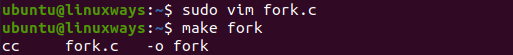And running the script, we get the result as below screenshot.

`\$ ./fork`### exec()

The exec() is such a system call that runs by replacing the current process image with the new process image. However, the original process remains as a new process but the new process replaces the head data, stack data,etc. It runs the program from the entry point by loading the program into the current process space.

To elaborate more, let’s take an example as shown below.

`\$ sudo vim exec.c`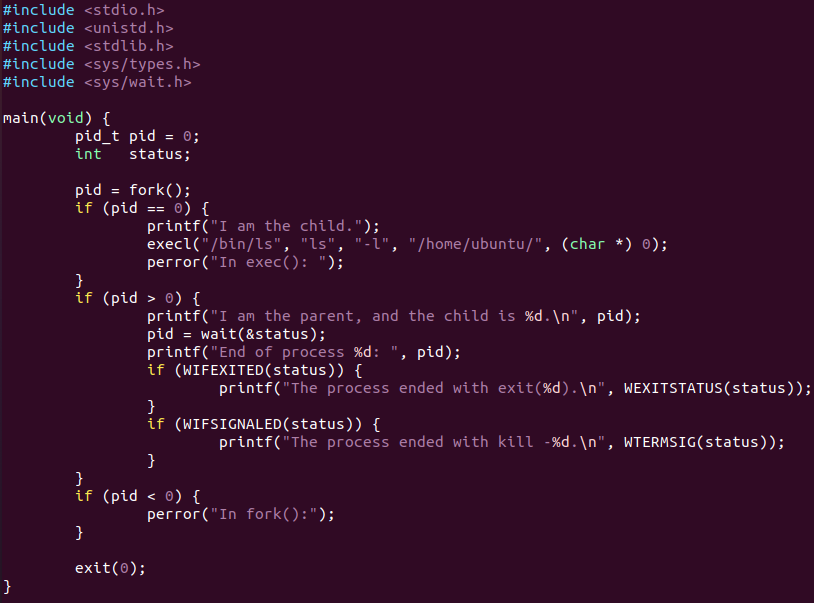And here is the code:

```#include <stdio.h>
#include <unistd.h>
#include <stdlib.h>
#include <sys/types.h>
#include <sys/wait.h>

main(void) {
pid_t pid = 0;
int status;
pid = fork();

if (pid == 0) {
printf("I am the child.");
execl("/bin/ls", "ls", "-l", "/home/ubuntu/", (char *) 0);
perror("In exec(): ");
}

if (pid > 0) {
printf("I am the parent, and the child is %d.\n", pid);
pid = wait(&status);
printf("End of process %d: ", pid);

if (WIFEXITED(status)) {
printf("The process ended with exit(%d).\n", WEXITSTATUS(status));
}

if (WIFSIGNALED(status)) {
printf("The process ended with kill -%d.\n", WTERMSIG(status));
}
}

if (pid < 0) {
perror("In fork():");
}

exit(0);
}```

Output:

`\$ make exec`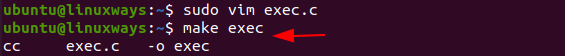And running the script, we get the result as below screenshot.

`\$ ./exec`### wait()

As in the case of a fork, child processes are created and get executed but the parent process is suspended until the child process executes. In this case, a wait() system call is activated automatically due to the suspension of the parent process. After the child process ends the execution, the parent process gains control again.

To elaborate about the wait(), let’s take an example which clarifies the wait() system call.

`\$ sudo vim wait.c`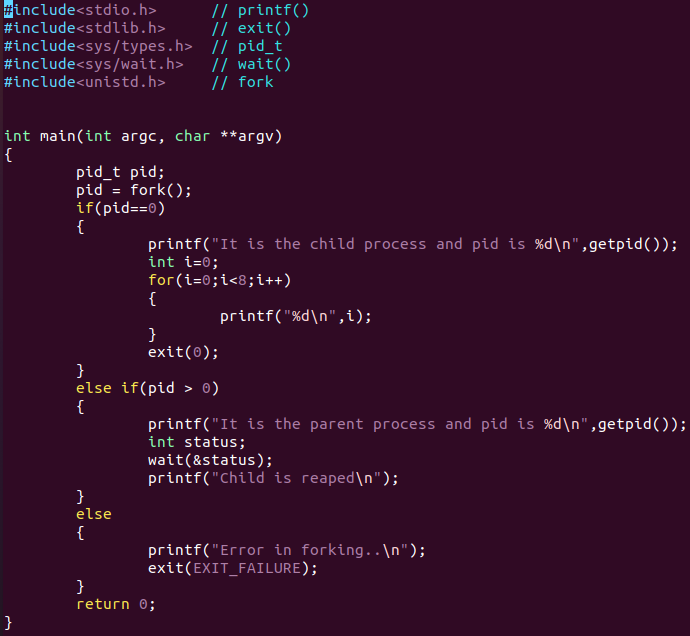An here is the code example:

```#include<stdio.h> // printf()
#include<stdlib.h> // exit()
#include<sys/types.h> // pid_t
#include<sys/wait.h> // wait()
#include<unistd.h> // fork

int main(int argc, char **argv)
{

pid_t pid;
pid = fork();

if(pid==0)

{
printf("It is the child process and pid is %d\n",getpid());

int i=0;
for(i=0;i<8;i++)
{
printf("%d\n",i);
}

exit(0);

}
else if(pid > 0)
{

printf("It is the parent process and pid is %d\n",getpid());

int status;
wait(&status);
printf("Child is reaped\n");
}

else
{

printf("Error in forking..\n");
exit(EXIT_FAILURE);

}

return 0;

}```

Output:

`\$ make wait`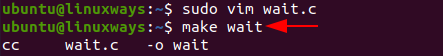And running the script, we get the result as below screenshot.

`\$ ./wait`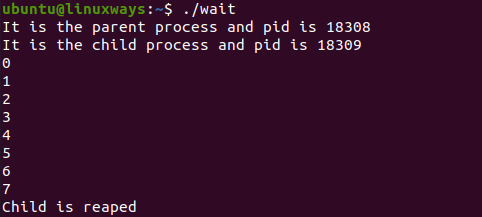### exit()

The exit() is such a function or one of the system calls that is used to terminate the process. This system call defines that the thread execution is completed especially in the case of a multi-threaded environment. For future reference, the status of the process is captured.

After the use of exit() system call, all the resources used in the process are retrieved by the operating system and then terminate the process. The system call Exit() is equivalent to exit().

Synopsis

```#include <unistd.h>
void _exit(int status);
#include <stdlib.h>
void _Exit(int status);```

You can see the use of exit() function on the above examples of fork(), wait(). The use of exit() system call is done to terminate the process.

### Conclusion

In this article, we learned the fork(), exec(), wait() and exit() system calls in detail with some examples. For more details, try running the programs by using those system calls and see the result. Thank you!

Fork, exec, wait and exit system call explained in Linux#### Karim Buzdar

About the Author: Karim Buzdar holds a degree in telecommunication engineering and holds several sysadmin certifications. As an IT engineer and technical author, he writes for various web sites. You can reach Karim on LinkedIn